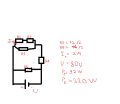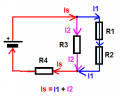Stuck on a resistor homework assignment

Human2

Joined Dec 22, 2017
51
Okay so this is the schematic:I need to calculate R1, R2, R5 and I.

I calculated R1 = 8 ohms and R5 = 20 ohms with the help of P1(I1) and P5(U).
I then got the current at the resistor R5 which is 4 A

Now I only have to calculate the current and R2, but I just cant I tried with a lot of equasions and failed can someone
slove it or at least point me to the right direction ? Thanks in advanced

Jony130

Joined Feb 17, 2009
5,292
For now you can remove R5 resistor from the circuit (do you know why) and you could try to apply KVL around the loop form by the supply voltage source and R3 and R4.
And keep in mind that IR4 = I1 + IR3

Last edited:
•Human2

Human2

Joined Dec 22, 2017
51
For now you can remove R5 resistor from the circuit (do you know why) and you could try to apply KVL around the loop form by the supply voltage source and R3 and R4.
And keep in mind that IR4 = I1 + IR3
Hmmm I dont know why I can remove R5 :/

And also: IR4 = I1 + IR3 -> U4 = I1 + I*R3 how did you get to that

Last edited:

Jony130

Joined Feb 17, 2009
5,292
Hmmm I dont know why I can remove R5 :/
Because R5 is in parallel with the voltage source. And in parallel circuits the two or more components are connected to the same nodes and, therefore, they have the exact same voltage across them.
So we can for now forget about R5 and solve the rest part of a circuit.

And also: IR4 = I1 + IR3 -> U4 = I1 + I*R3 how did you get to that

Try analysis the current path•Human2

Human2

Joined Dec 22, 2017
51
I know that I12 + I3 = I4 and that I4 = I,
I know that U12 = U3 and that U = U12 + U4
I know that (U12/R12) + (U3/R3) = (U4/R4)
I know that I3 * R3 + I*R4 = I*R
I know that I12 * R12 + I*R4 = I*R

but I just don't see I*R4 = I12 + I * R3 :/

LesJones

Joined Jan 8, 2017
3,661
Start by considering R1 + R2 as one resistor. (Call it Rx) So now you have Rx in parallel with R3 and these are in series with R4. You know the voltage between the ends of this network is 80 volts . You don't know the current through R3 so call it Ix. You know the current through Rx. Write down the equations for the voltage drop across R4 (Call this Vx), Rx and R3 Fill in the values for the things you know. You should then be able to eliminate one of the unknowns by combinine these equations to solve for Ix. You will only really use two of these equations.
Another method would be to use Thevenins theorem.

Les.

•Human2

Jony130

Joined Feb 17, 2009
5,292
but I just don't see I*R4 = I12 + I * R3 :/
I see the confusion.
This

IR4 = I1 + IR3

It is not equal to IR4 = I*R4 = U4 wrong.

What I ment is this
IR4 - current through R4 resistor
IR3 - current through R3 resistor

And U4 = IR4*R4

So,

I4 = I12 + I3 when I use your notation.

Is this clear now?

If so, please write a II Kirchhoff's law equation in the loop U, R3 and R4 in terms of a current and solve for I3

Last edited:
•Human2

Human2

Joined Dec 22, 2017
51
Thanks I got it I inserted I3 = I4 - 2 in voltaage equation U4 = U - U3 and got that current...
I spent 1 hour on this I was trying ot do it over ressistance got cubic equation it was a mess

Human2

Joined Dec 22, 2017
51
I see the confusion.
This

IR4 = I1 + IR3

It is not equal to IR4 = I*R4 = U4 wrong.
Ooohh hah it got me confused, I see now.. thanks for helping guys

Jony130

Joined Feb 17, 2009
5,292

Human2

Joined Dec 22, 2017
51
I3 is 2, I4 = 8, R2 = 4 ohms

oh and then I is 4(currenct through R4) + 4(current through R5) = 8 A

•Jony130

WBahn

Joined Mar 31, 2012
26,398
R

but I just don't see I*R4 = I12 + I * R3 :/
Which is good, because it can't. I*R4 is a voltage, as is I*R3. But I12 is a current and you cannot add voltages and currents, any more than you can add kilograms and kilometers.

•Human2